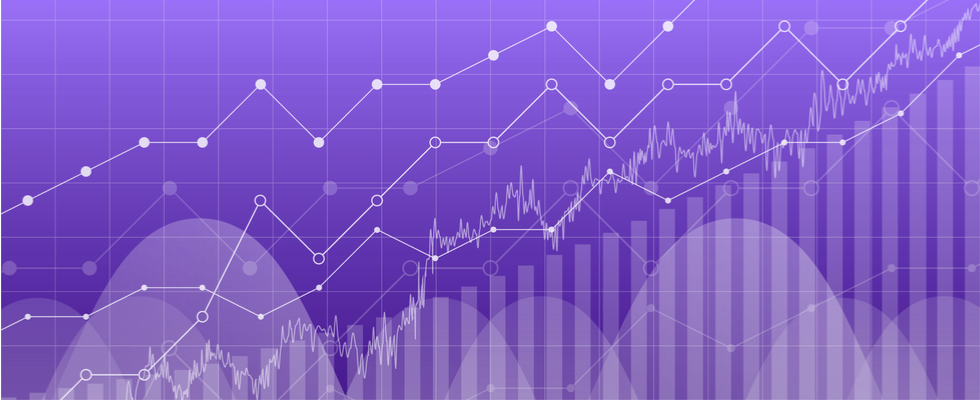# Tag: Matplotlib

## Python Drawing: Intro to Python Matplotlib for Data Visualization (Part 2)Ever wondered how you can use Python to create stunning data visualizations? In the first part of this series, we saw how to draw line plots and histograms using the matplotlib library. We also saw how to change the default size of a plot and how to add titles, axes, and legends to a plot. In this article, we’ll see a few more types of plots that can be drawn using the matplot library with pro tips on how to improve them.

## Python Drawing: Intro to Python Matplotlib for Data Visualization (Part 1)

Want to know how Python is used for plotting and data visualization? Interested in learning one of the most commonly used data visualization libraries in Python? If so, you’re in the right place. In this installment of a two-part tutorial, we’ll learn how to use matplotlib, one of the most commonly used data visualization libraries in Python. Over the course of both articles, we’ll create different types of graphs, including: